Next: Sawtooth wave Up: Classical waveforms Previous: Dissecting classical waveforms   Contents   Index

Fourier series of the elementary waveforms

In general, given a repeating waveform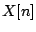, we can evaluate its Fourier series coefficients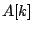by directly evaluating the Fourier transform: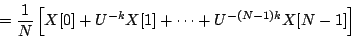but doing this directly for sawtooth and parabolic waves will require pages of algebra (somewhat less if we were willing resort to differential calculus). Instead, we rely on properties of the Fourier transform to relate the transform of a signal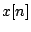with its first difference, defined as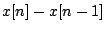. The first difference of the parabolic wave will turn out to be a sawtooth, and that of a sawtooth will be simple enough to evaluate directly, and thus we'll get the desired Fourier series.

In general, to evaluate the strength of theth harmonic, we'll make the assumption that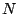is much larger than, or equivalently, thatis negligible.

We start from the Time Shift Formula for Fourier Transforms (Page) setting the time shift to one sample:Here we're using the assumption that, becauseis much larger than,is much smaller than unity and we can make approximations:which are good to within a small error, on the order of. Now we plug this result in to evaluate:SubsectionsNext: Sawtooth wave Up: Classical waveforms Previous: Dissecting classical waveforms   Contents   Index
Miller Puckette 2006-12-30